Pressure: force divided by area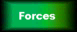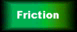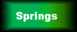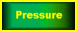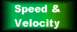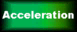Pressure depends on two things:

• the Force (in Newtons) and

• the Area it's pressing on (in square metres)
 We work it out using: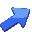Pressure = Force ÷ Area Pressure is measured in Pascals (Pa for short),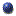1 Pascal means 1 Newton per square metreNamed after Blaise Pascal (1623- 1662), a French mathematician and physicist
 Here's an example: the force on the bench is the weight of the block: 80 N The area it's pressing on is the base area of the block: 2 square metres So the pressure on the bench is 80 ÷ 2 = 40 Pascals

Notice that a large force might only create a small pressure if it's spread out over a wide area.
Also, a small force can create a big pressure if the area is tiny.

Try these examples yourself (you may need a calculator):

1) An office safe has a weight of 500N. If the area of the base is 1.25 square metres, what is the pressure on the floor of the office?

2) A physics teacher has a weight of 800N. If his feet have an area of 0.025 square metres each, what pressure does he exert on the ground? (Remember, he has two feet!)

At GCSE level, you're unlikely to be asked anything more complex than these two questions.

Gases create a pressure on their containers, because of the gas molecules colliding with the walls.

Using Pressure

• When the area is small, a moderate force can create a very large pressure. This is why a sharp knife is good at cutting things: when you push the very small area of the sharp blade against something, it creates a really large pressure.

• Ice skates have sharp edges, and thus a small area in contact with the ice.
This means that your weight creates a very large pressure on the ice, far more than if you were standing in ordinary shoes.
Ice has an unusual property: it can melt under pressure, even if it's below 0°C. When you're ice skating, you're actually skating on a layer of water that you've just melted, which quickly re-freezes when you move on (you're not skating on ice at all!) This is called regelation, and means that there's very little friction as you skate along.

• Even a slender supermodel can damage floors by walking on then in high-heeled shoes. This is because the area of the heel is small, so you can easily create enough pressure to cause a dent in the floor.
The pressure can be greater than if an elephant was standing there, even though the force is much less. So you should be able to figure out why elephants and camels have large feet.
 As you go deeper into a liquid, the pressure increases. You can feel this on your ears as you swim down to the bottom of a swimming pool. We need to remember this when designing the walls of pools and dams: the wall must be thicker at the bottom, to withstand the increased pressure down there.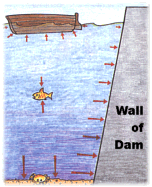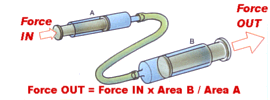We can use pressure in liquids to move a piston and do useful work. This is how the hydraulic systems in diggers, car brakes and fairground rides work: a pump creates pressure in an incompressible liquid, which acts on a piston. By adjusting the area of the piston, we can adjust the force we get.

• The pressure of the atmosphere on you right now is around 100,000 Pa. OK, that's just a number, so think of it this way:
- you probably have a skin area of around 2 square metres,
- Pressure = force ÷ area, so force = pressure x area
thus the force on you = 100,000 x 2 = 200,000 Newtons.
That's about the same force as having over a dozen cars piled on top of you!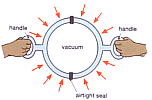Otto von Guericke, (1602-1686), a German physicist, born in Magdeburg, performed a famous experiment: the "Magdeburg Hemispheres". These were two halves of a large, hollow metal ball. When all the air was sucked out of the ball, two teams of eight horses couldn't pull them apart, because the pressure of the Earth's atmosphere created a very large force on the ball. You may have seen a smaller version of this experiment in school.

Questions:

3) Have you figured out why camels have large feet yet?

4) In an hydraulic car braking system, why do we make the piston down by the wheel BIGGER than the piston by the brake pedal?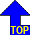of page